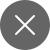# How does a confusion matrix work in classification models?

4+

The confusion matrix is basically used to know the performance measurement of a trained(fit) classification model. It tells the Data Scientists as to where they stand with respect to the number of classifications or number of predictions they’ve made correctly or not.

### What is a confusion matrix?

As I’ve told above it is mainly a performance measure, a measure of utmost importance in the classification models of machine learning, such as Logistic Regression, Naive Bayes, SVM, etc, like Organisational Behavior’s Jo Hari Window, it is a table with 4 different combinations or categories of predicted values or actual values. Refer to the image below:

Let us go a bit inside the window as to what are TP, FP, FN, & TN.

TP – Stands for True Positive, i.e whatever the classification model predicted, it predicted correctly. For example, if the model predicted that the tumor is malignant, it is malignant.

TN – Stands for True Negative, for example, if the tumor is not malignant, it is not.

FP – Stands for False Positive, for example, the tumor is predicted malignant, but it is not. It is also known as a Type I error.

FN – Stands for False Negative, for example, the tumor is predicted not malignant, but actually is, also known as a Type II error.

### Calculation of a confusion matrix

A confusion matrix is very much useful in the calculation of accuracy, precision, recall, and AOC-ROC Curve(will be explained in the next article).

Refer the image below for the math behind the confusion matrix:

Let us take a look at the working shown in the above image. We will look at the output for threshold, which is equal to 0.6, the threshold can be taken as a median of a set of y pred(y predicted) values. The predicted values which are greater than 0.6 will be denoted as 1 and less than 0.6 will be denoted as 0.

#### Formula for Recall:

TP in the above case is equal to 2, refer the image above as it shows that there are 2 values equal to y(actual value) and y pred(predicted value). FN is also equal to 2, as there are three values that are different than the actual and predicted. So, in this case, Recall will be equal to 1/2.

#### Formula for Precision:

TP is equal to 2 and FP is equal to 1. Refer to the image above. Hence, precision is equal to 2/3.

#### Accuracy:

It can be calculated as the Total Number of Right Predictions/Total Number of Values, which is equal to 4/7.

#### Formula for F1 Score:

Sometimes, due to high precision & low recall & vice versa, it comes difficult to compare them both, so in order to keep both the measurements comparable, F1 score or F- the measure is used. It uses the harmonic mean.

#### Syntax of a confusion matrix using python:

We will start importing the necessary metrics related to confusion matrix, precision, recall, etc, ignore Logistic Regression & train, test split, that we will discuss in another article.

Syntax of confusion matrix using python:

We are the values of the test data set of y with the predicted values of y, in the above screenshot of the code.

Creating a heat map of the confusion matrix: